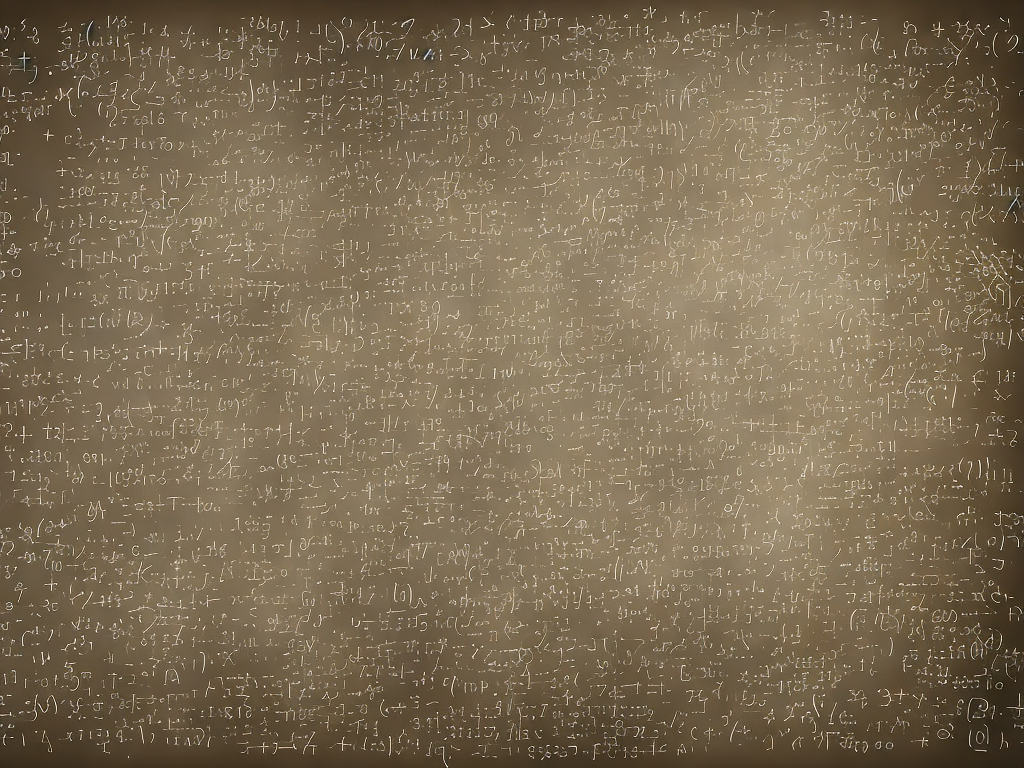# Half Of The Difference Between Two Numbers Is 2. The Sum Of The Greater Number And Twice The Smaller Number Is 13. Find The Numbers.

tl;dr
The two numbers are 7 and 3, which can be found by translating the given information into algebraic equations and solving them.When you are facing numbers in algebra problems, the first step is always to read the problem statement carefully and identify the key information provided. In this case, we have two numbers, and we know that "Half of the difference between two numbers is 2." Let's call the greater number "x" and the smaller number "y."

Using algebra, we can write the statement above as:

(x - y) / 2 = 2

We can simplify this equation by multiplying both sides by 2:

x - y = 4

This equation tells us that the difference between the two numbers is 4. However, we still don't know what the actual numbers are. We need more information to solve the problem.

The second statement we have is: "The sum of the greater number and twice the smaller number is 13." We can write this as an algebraic equation too:

x + 2y = 13

Now we have two equations with two variables, and we can solve for x and y.

One way to solve this system of equations is by substitution. We can solve one equation for one variable and substitute it into the other equation to eliminate that variable. For example, we can solve the first equation for x:

x = y + 4

Now we can substitute this expression for x in the second equation:

(y + 4) + 2y = 13

Simplifying this equation, we get:

3y + 4 = 13

Subtracting 4 from both sides of the equation, we obtain:

3y = 9

Dividing both sides by 3, we find that:

y = 3

Now that we know the value of y, we can use the equation x = y + 4 to find the value of x:

x = 3 + 4 = 7

Therefore, the two numbers are 7 and 3. We can check our answer by verifying that the two statements we were given are true for these numbers:

Half of the difference between 7 and 3 is 2:

(7 - 3) / 2 = 2

The sum of 7 and twice 3 is 13:

7 + 2(3) = 13

Therefore, we can conclude that the numbers are indeed 7 and 3.

In conclusion, this problem demonstrates how we can use algebra to solve real-world problems involving numbers. By carefully reading the problem statement, identifying the key information provided, and translating that information into algebraic equations, we can find the solution to the problem. In this case, we found that the two numbers were 7 and 3, which satisfied the conditions given in the problem statement.